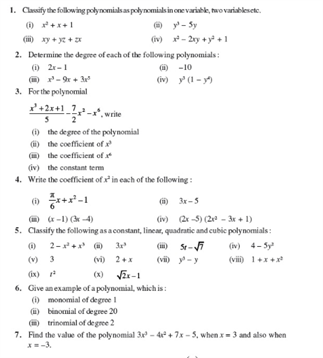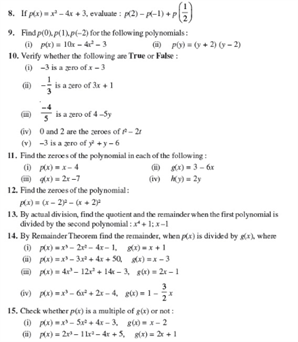# Revision Notes For CBSE Class 9 Math Chapter -2 Polynomials

An expression comprising of more than two algebraic terms are referred to as a polynomial. They are the sum of different terms containing different powers of variables.

A polynomial can have many terms including:

• Constants such as 1, 2, 3….
• Variables such as g, h, x, y…..
• Exponents such as 5 in x5 ……

Polynomials often represent a function. The term Polynomials is derived from a Greek word which refers to the multiple terms –The word Poly refers to multiple and Nominal refers to terms. This mathematical expression is constructed with constants and variables. Monomials, Binomials, Trinomials are different types of polynomials.

Check here for the free CBSE Class 9 Math revision notes for Chapter 2- Polynomials